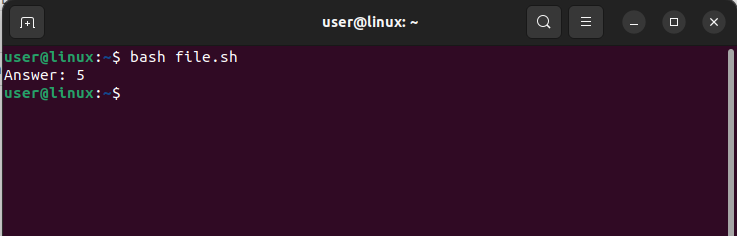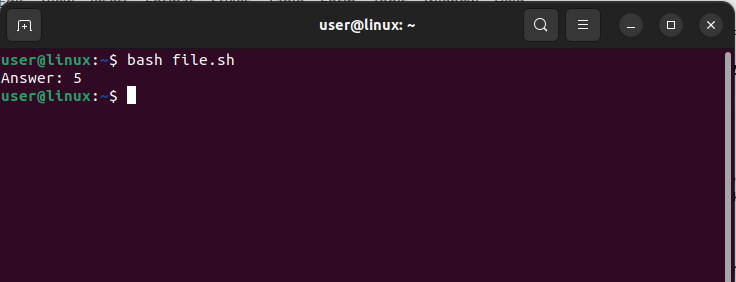# How to Divide Two Variables in Bash

Working with variables in Bash often involves performing mathematical operations, such as division. While dividing two variables may seem straightforward, it requires careful handling in the Bash scripting language. This article serves as a detailed guide, providing examples to demonstrate the proper approach to dividing variables in Bash and how to incorporate this operation into your scripts.

## How to Divide Two Variables in Bash

You can divide two variables in Bash using:

• Double Parentheses
• expr Command

### Method 1: Using the Double Parentheses

Here is an example code to divide two variables using the double parentheses syntax, which is a simplified technique to execute arithmetic operations in Bash:

 ```#!/bin/bash # Declare variables var1=10 var2=2 # Divide variables using double parentheses syntax Answer=\$((var1 / var2)) echo "Answer: \$Answer"```

In this code, we utilize the double parentheses syntax to divide the value of var1 by the value of var2. The quotient obtained from this division operation is assigned to the result variable, and subsequently, it is displayed on the console.### Method 2: Using the expr Command

The expr command in Bash is employed to evaluate an expression and display the resulting value on the console. If you wish to divide two variables using the expr command, consider the following code example:

 ```#!/bin/bash # Declare variables var1=10 var2=2 # Divide variables using expr command Answer=\$(expr \$var1 / \$var2) echo "Answer: \$Answer"```

In this specific illustration, the expr command is employed to divide the value of var1 by the value of var2. Subsequently, the outcome of this division operation is captured in the result variable and subsequently displayed on the console.## Conclusion

When dealing with numerical data in Bash, it is frequently necessary to divide two variables. This article explores two commonly used techniques for achieving this task. By employing either the expr command or the double parentheses syntax, you can effortlessly and efficiently divide two variables within your Bash scripts.